# Lab 8: Spacing and distances

This session is concerned with summary statistics for spacings and interpoint distances. The lecturer’s R script is available here (right click and save).

``````library(spatstat)
``````

### Exercise 1

For the `swedishpines` data:

1. Calculate the estimate of the nearest neighbour distance distribution function G using `Gest`.

``````G <- Gest(swedishpines)
``````
2. Plot the estimate of G(r) against r

``````plot(G, cbind(km, rs, han) ~ r, main = "Nearest neighbor distance distribution")
``````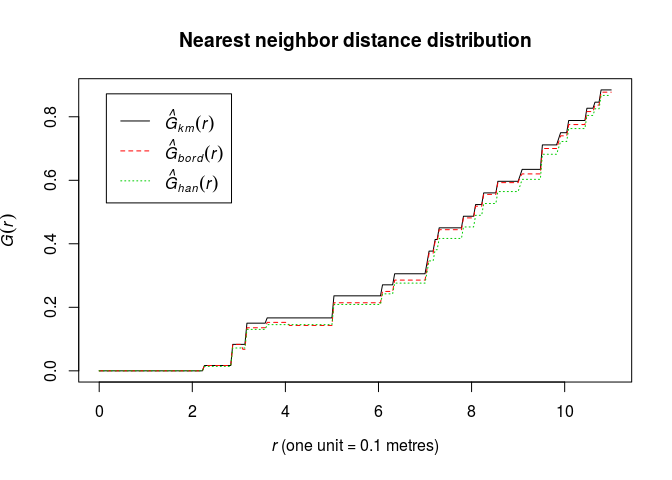3. Plot the estimate of G(r) against the theoretical (Poisson) value Gpois(r)=1 − exp(−λπr2).

E.g.

``````plot(G, . ~ theo, main = "Nearest neighbor distribution")
``````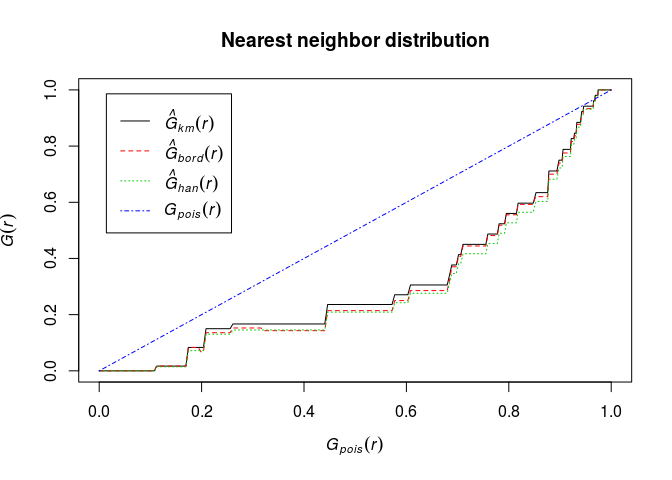4. Define Fisher’s variance-stabilising transformation for c.d.f.’s by

``````Phi <- function(x) asin(sqrt(x))
``````

Plot the G function using the formula `Phi(.) ~ Phi(theo)` and interpret it.

``````Phi <- function(x) asin(sqrt(x))
plot(G, Phi(.) ~ Phi(theo), main = "Nearest neighbor distribution")
``````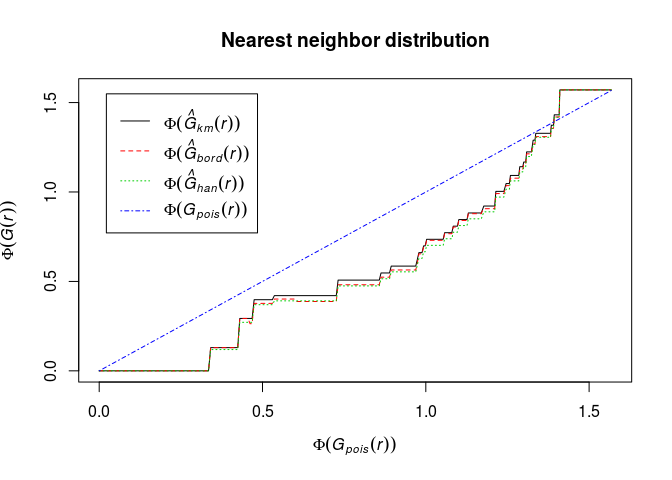The transformation has made the deviations from CSR in the central part of the curve smaller. We need envelopes to say anything about significance.

### Exercise 2

For the `swedishpines` data:

1. Calculate the estimate of the nearest neighbour distance distribution function F using `Fest`.

``````Fhat <- Fest(swedishpines)
``````
2. Plot the estimate of F(r) against r

``````plot(Fhat, main = "Empty Space function")
``````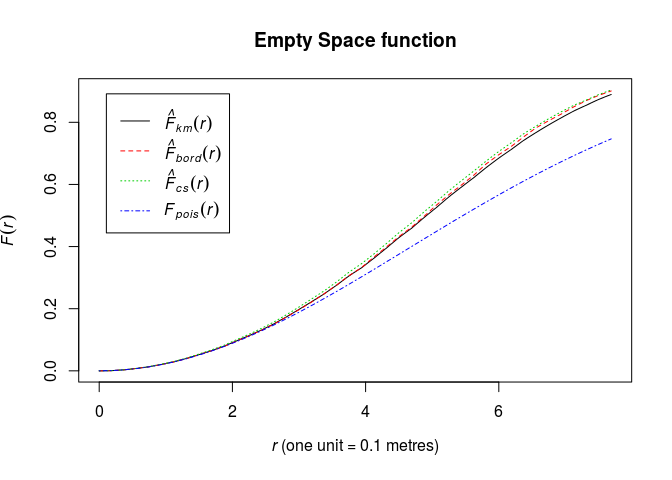3. Plot the estimate of F(r) against the theoretical (Poisson) value Fpois(r)=1 − exp(−λπr2).

``````plot(Fhat, . ~ theo, main = "")
``````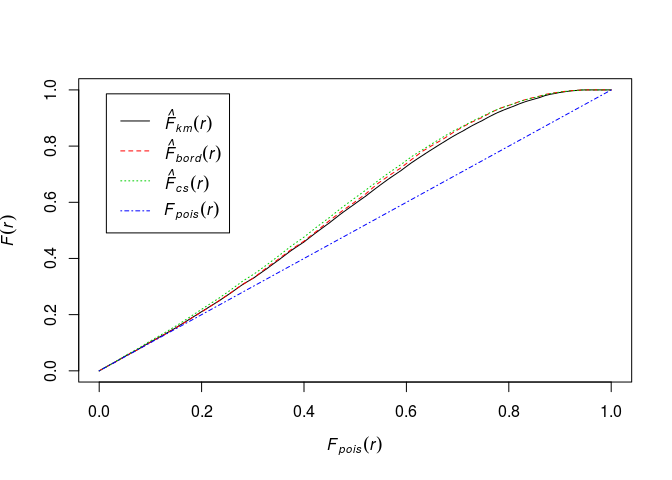4. Define Fisher’s variance-stabilising transformation for c.d.f.’s by

``````Phi <- function(x) asin(sqrt(x))
``````

Plot the F function using the formula `Phi(.) ~ Phi(theo)` and interpret it.

``````Phi <- function(x) asin(sqrt(x))
plot(Fhat, Phi(.) ~ Phi(theo), main = "")
``````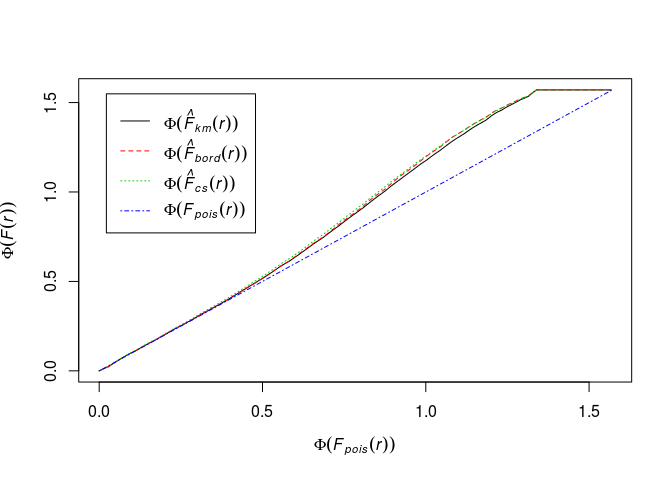The transformation has changed the picture much. It looks like deviation from CSR, but again, without envelopes it’s hard to draw conclusions.